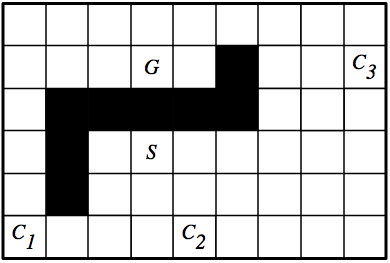Assignment One: Search
Due: 12:30pm, Monday 25 September 2006

Some of the following problems will be easier to do if you use the CIspace applets at
http://www.cs.ubc.ca/labs/lci/CIspace/

# Question One

Consider the grid world in the following figure:Assume that the robot can be in any of the white squares, and can do one step up, down, left, or right at each time. It cannot step into one of the black squares or outside of the boundary. The cost of a path is the number of steps in the path. At the squares marked with Ci are coffee shops where, if the robot goes to the square, it will be given coffee. Suppose the robot starts at the square marked as S, without coffee, and must end up at the square marked G carrying coffee.
1. What is the state space? How many states are there?
2. Draw the graph that represents the search space. You can use whatever notation you like as long as you explain it.
3. Give an admissible heuristic. Your heuristic can take into account where the coffee shops are, but not where the blocked squares are. Hint: a useful concept is the Manhattan distance between two points (x1,y1) and (x2,y2) which is |x1-x2|+|y1-y2|.
4. On your graph number the nodes in the order they are expanded in an A* search with multiple path pruning.
5. How will your heuristic and A* change if we assume that there is a hill going down towards the right so that steps to the right cost 0.5 and steps to the left cost 1.5, and steps up and down cost 1 each.

# Question Two

Progress is made in science by observing a phenomenon of interest, making hypotheses and testing the hypothesis either empirically or by proving theorems.

For this question you are to think about the effect of heuristic accuracy on A* search. That is, you are to experiment with, and think about how close h(n) is to the actual distance from node n to a goal affects the efficiency and accuracy of A*. To get full marks you must at least invent and prove one theorem and show some empirical evidence for you answer. Your answers need to be precise (e.g, don't say "it works better", but say something like "it always works better", "it sometimes works better" or "it works better in a majority of cases").

1. What is the effect of reducing h(n) when h(n) is already an underestimate?
2. How does A* perform when h(n) is the exact distance from n to a goal?
3. What happens if h(n) is not an underestimate?
One way to vary h(n) is to use the applet with the automatic node heuristics (under the search options menu) turned on, then turn it off and change heuristic values. There are ways to change all heuristic values.

# Question Three

For each of the following, give a graph that is a tree (there is at most one arc into any node), contains at most 15 nodes, and has at most two arcs out of any node.
1. Give a graph where depth-first search is much more efficient (expands fewer nodes) than breadth-first search.
2. Give a graph where breadth-first search is much better than depth-first search.
3. Give a graph where A* search is more efficient than either depth-first search or breadth-first search.
4. Give a graph where depth-first search and breadth-first search are both more efficient than A* search.
You must draw the graph and show the order of the neighbors (this is needed for the depth-first search). Either give the arc costs and heuristic function or state explicitly that you are drawing the graph to scale and are using Euclidean distance for the arc costs and the heuristic function.